: How students discovered Pascal : pascal like triangles : Contents   Contents

# Abstract

We can make Pascal like triangles by the list of probabilities based on a game, and we can produce some beautiful Sierpinski like Gaskets using these triangles.

These triangles are generalizations of the well known Pascal's triangle, and these Gaskets can be considered as generalizations of the well known Sierpinski Gasket.

If you are interested in beautiful mathematical structure, please look at beautiful pictures of Pascal like triangles and Sierpinski like gaskets in Section 5. Perhaps you can appreciate the beauty figures that were produced by mathematical structures.

Let us illustrate the game that we are going to use in this article. Letbe fixed natural number such that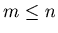. We have players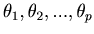who are seated around a circle, and we are going to choose one loser of the game. The game begins with player. Proceeding in order, a box is passed from hand to hand. The box contains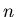numbers. The numbers in the box are assigned in numerical order, from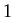to. When a player receives the box, he draws a number from the box. The players cannot see the numbers when they draw them, and hence they draw at random. Once a certain number is taken from the box, that number will not be returned to the box. If any player gets a numbersuch that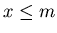, he will be the loser and the game ends.

We denote bythe probability of theth player's losing in this game. Then the listandforms a Pascal like triangle of fractions, and from this triangle we can make Sierpinski like Gasket.

These new facts of mathematics were discovered by a group of high school students and a high school teacher who worked with them. At first students studied the game of Russian roulette, and discovered facts. Later the authors (students and the teacher) made a mathematically same game to use in this article, because some people may think that Russian roulette is not a good topic to study.

The high school teacher is Dr.Miyadera who is a mathematician. Although he helped students to prove theorems, most of the important facts were discovered by high school students.

This article presents some interesting facts of mathematics, and at the same time this article presents an example of research by high school students and the possibility of new way of teaching how to be creative in mathematics.: How students discovered Pascal : pascal like triangles : Contents   Contents